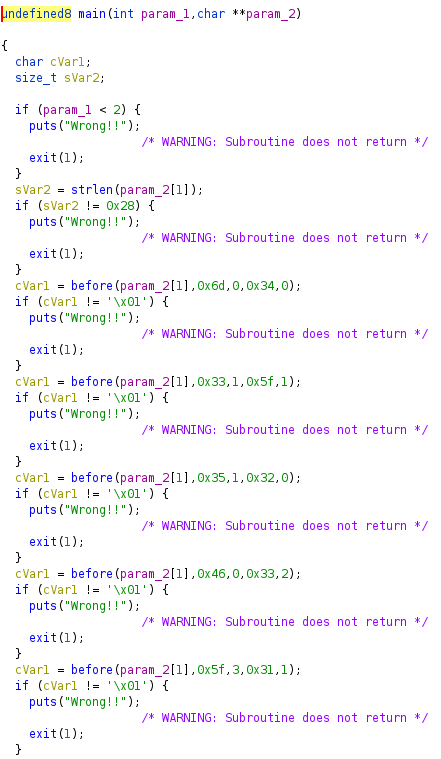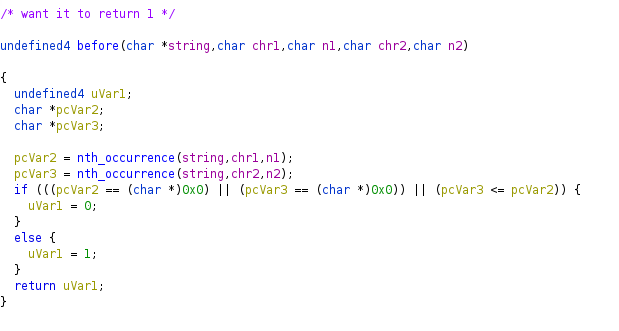Welcome to the Debugmen blog. We use this site to post tools, security findings, CTF writeups and anything else we find worthy of release to the public.

# well-ordered

## Overview

This challenge hands you a x86 ELF. Based on the other RE challenges in this CTF it probably takes input as a command line argument.

Lets open it in ghidra and see what it’s doing. Below is the Ghidra decompilation of `main`Looks like it reads input from `argv` and does a ton of checks. What you see here is what the rest of the main function looks like. There are 42 checks; the first checks if enough command line arguments are supplied and the second checks if `argv`’s length is 40. The rest of the 40 checks all call `before()``before()` checks if the nth occurence of one character appears before the kth occurence of another character (for some n and k). If that is the case, then return 1, otherwise 0`nth_occurence()` returns a pointer to the nth character appearing in the string. Here, n = 0 means the first occurance. Note that this funcion is recursive.

If it wasn’t obvious from the function names, this program just checks a series of constraints on the user input based on the nth occurrence of a letter appearing before the kth occurence of another letter.

## Angr Attempt 1

Since there are a bunch of contraints (and I’m lazy), my first thought is to write an angr script and call it a day

``````import angr
import claripy
import IPython

filename = 'well-ordered'
base = 0
proj = angr.Project(filename, main_opts={'base_addr':base})
cfg = proj.analyses.CFGEmulated(keep_state=True)

goal_addr = base+ 0x1b61
avoid_addr = base + 0x12af

arg1 = claripy.BVS('arg', 128*8)

entry_state = proj.factory.entry_state(args=[filename, arg1])
simgr = proj.factory.simulation_manager(entry_state)

IPython.embed()
``````

Unfortunately, this never finished running until it ate all of my VM’s memory and died. At first I thought the recursion was destroying performance and causing angr to run out of memory storing all the states, so that leads me to my second attempt trying to make this work.

## Angr Attempt 2

I thought it was the recursion that was killing angr’s performance, so I rewrote the challenge program such that `nth_occurence()` worked iteratively

``````#include <stdio.h>
#include <stdlib.h>
#include <string.h>

int before(char* str, char c1, char n1, char c2, char n2)
{
// Get the index of the n1-th occurance of c1
// Get the index of the n2-th occurance of c2
// Check if the first index is less than the second
int len = strlen(str);
char* tmp = str;
char* pos1;
char* pos2;

for(int i = n1; i >= 0; i--)
{
pos1 = strchr(tmp, (int) c1);
if(pos1 == NULL) return 0;
tmp = pos1 + 1;
}

tmp = str;
for(int i = n2; i >= 0; i--)
{
pos2 = strchr(tmp, (int) c2);
if(pos2 == NULL) return 0;
tmp = pos2 + 1;
}
if (pos1 < pos2)
return 1;
return 0;

}

{
printf("%d\n", x);
exit(1);
}
int main(int argc, char** argv)
{
if(argc < 2) bad(-1);
if(strlen(argv) != 0x28) bad(0);

if(!before(argv, 'm', 0, '4', 0)) bad(1);
if(!before(argv, '3', 1, '_', 1)) bad(2);
if(!before(argv, '5', 1, '2', 0)) bad(3);
if(!before(argv, 'F', 0, '3', 2)) bad(4);
if(!before(argv, '_', 3, '1', 1)) bad(5);
if(!before(argv, '3', 2, '_', 3)) bad(6);
if(!before(argv, '_', 0, '5', 0)) bad(7);
if(!before(argv, 'y', 0, '0', 0)) bad(8);
if(!before(argv, '0', 2, 'e', 0)) bad(9);
if(!before(argv, 'R', 0, '_', 2)) bad(10);
if(!before(argv, 'r', 0, '3', 1)) bad(12);
if(!before(argv, 'c', 0, '5', 1)) bad(13);
if(!before(argv, 's', 0, '_', 4)) bad(14);
if(!before(argv, 'k', 0, '3', 0)) bad(15);
if(!before(argv, 'a', 0, '8', 0)) bad(16);
if(!before(argv, 'N', 0, '_', 5)) bad(17);
if(!before(argv, '1', 0, 'F', 0)) bad(18);
if(!before(argv, '_', 1, 'y', 0)) bad(19);
if(!before(argv, '8', 0, 'c', 0)) bad(20);
if(!before(argv, 'R', 1, '_', 6)) bad(21);
if(!before(argv, '1', 1, 's', 0)) bad(22);
if(!before(argv, 'l', 0, '1', 0)) bad(23);
if(!before(argv, '3', 0, '_', 0)) bad(24);
if(!before(argv, 'e', 0, 'e', 1)) bad(25);
if(!before(argv, '2', 0, '0', 2)) bad(26);
if(!before(argv, '0', 1, 'R', 1)) bad(27);
if(!before(argv, '_', 5, '0', 1)) bad(28);
if(!before(argv, 'd', 0, '3', 3)) bad(29);
if(!before(argv, '_', 2, 'l', 0)) bad(30);
if(!before(argv, '5', 0, 'U', 0)) bad(31);
if(!before(argv, 'i', 0, 'N', 0)) bad(32);
if(!before(argv, '_', 6, 'a', 0)) bad(33);
if(!before(argv, '0', 0, 'u', 0)) bad(34);
if(!before(argv, '3', 3, 'R', 1)) bad(35);
if(!before(argv, '4', 0, 'k', 0)) bad(36);
if(!before(argv, '_', 4, 'i', 0)) bad(37);
if(!before(argv, 'U', 0, 'r', 0)) bad(38);
if(!before(argv, 'r', 1, 'd', 0)) bad(39);
if(!before(argv, 'u', 0, 'R', 0)) bad(40);

puts("yay");
return 0;
}
``````

So I adjusted my angr script above so my goal address was the `puts("yay");` and the avoid was `bad()`. Yeah, this also didn’t work. It just kept running forever. Ok, maybe no angr

## I Have Brain Damage

At this point I have brain damage

Ok, so thinking more about this contraint problem made me realize that it’s kind of like one of those shitty leet code interview problems where you need to shuffle things around in an array to fit some constraint.

I wrote a python script that generates all the letters that exist in the string and starts swapping the positions of the letters specified in each constraint. That ended up working. This took considerably less time that everything else above 😔😔😔

For a given letter, taking the largest occurence specified in the contraints tells you how many times that letter appears in the string, and we also know that the string is 40 long. Then until all the constraints are satisfied, keep swapping the positions of letters that don’t satisfy the constraint tested.

``````constraints = [('m','\0'),('4','\0'),
('3','\x01'),('_','\x01'),
('5','\x01'),('2','\0'),
('F','\0'),('3','\x02'),
('_','\x03'),('1','\x01'),
('3','\x02'),('_','\x03'),
('_','\0'),('5','\0'),
('y','\0'),('0','\0'),
('0','\x02'),('e','\0'),
('R','\0'),('_','\x02'),
('r','\0'),('3','\x01'),
('c','\0'),('5','\x01'),
('s','\0'),('_','\x04'),
('k','\0'),('3','\0'),
('a','\0'),('8','\0'),
('N','\0'),('_','\x05'),
('1','\0'),('F','\0'),
('_','\x01'),('y','\0'),
('8','\0'),('c','\0'),
('R','\x01'),('_','\x06'),
('1','\x01'),('s','\0'),
('l','\0'),('1','\0'),
('3','\0'),('_','\0'),
('e','\0'),('e','\x01'),
('2','\0'),('0','\x02'),
('0','\x01'),('r','\x01'),
('_','\x05'),('0','\x01'),
('d','\0'),('3','\x03'),
('_','\x02'),('l','\0'),
('5','\0'),('U','\0'),
('i','\0'),('N','\0'),
('_','\x06'),('a','\0'),
('0','\0'),('u','\0'),
('3','\x03'),('R','\x01'),
('4','\0'),('k','\0'),
('_','\x04'),('i','\0'),
('U','\0'),('r','\0'),
('r','\x01'),('d','\0') ,
('u','\0'),('R','\0')]

freq = {}
for c in constraints:
c = (c, ord(c))
count = freq.get(c, 0)

if c >= count:
count = c
freq[c] = count

population = ""
for k, v in freq.items():
population += k*(v+1)

text = list(population)

def nth(text, char, n):
count = 0
for i in range(len(text)):
if text[i] == char:
count += 1
if count == n + 1:
return i

raise Exception

good = False
while not good:
good = True
for i in range(len(constraints)//2):
first = constraints[i*2]
second = constraints[i*2+1]

pos1 = nth(text, first, ord(first))
pos2 = nth(text, second, ord(second))

if pos1 >= pos2:
good = False
print(i, first, second)
tmp = text[pos1]
text[pos1] = text[pos2]
text[pos2] = tmp

print(''.join(text))
``````

`uscg{m4k3_5Ur3_y0uR_l1F3_1s_iN_0rd3R_a8c520ee}`